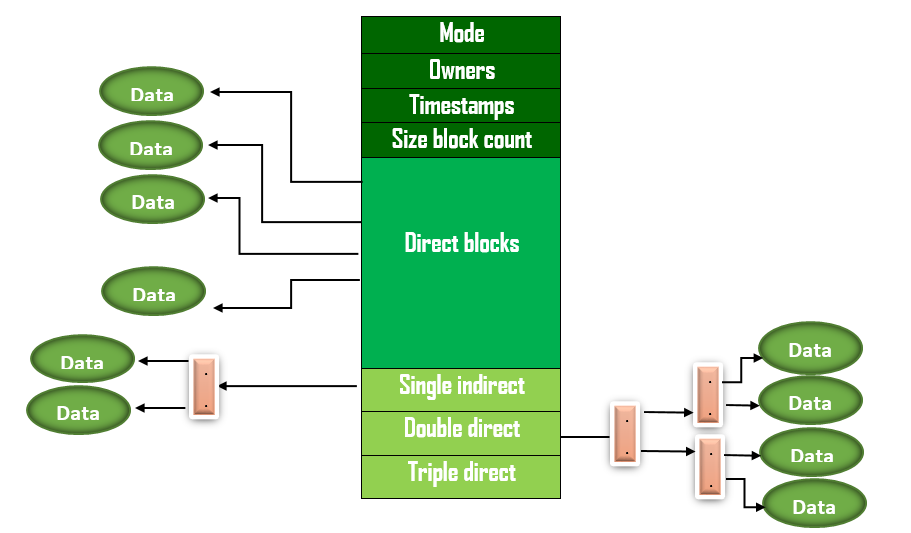# Operating Systems | Set 10

• Difficulty Level : Medium
• Last Updated : 13 Apr, 2018

Following questions have been asked in GATE 2008 CS exam.

1) The data blocks of a very large file in the Unix file system are allocated using
(A) contiguous allocation
(C) indexed allocation
(D) an extension of indexed allocation

The Unix file system uses an extension of indexed allocation. It uses direct blocks, single indirect blocks, double indirect blocks and triple indirect blocks. Following diagram shows implementation of Unix file system.2) The P and V operations on counting semaphores, where s is a counting semaphore, are defined as follows:

```P(s) : s =  s - 1;
if (s  < 0) then wait;
V(s) : s = s + 1;
if (s <= 0) then wakeup a process waiting on s;
```

Assume that Pb and Vb the wait and signal operations on binary semaphores are provided. Two binary semaphores Xb and Yb are used to implement the semaphore operations P(s) and V(s) as follows:

```P(s) : Pb(Xb);
s = s - 1;
if (s < 0) {
Vb(Xb) ;
Pb(Yb) ;
}
else Vb(Xb);

V(s) : Pb(Xb) ;
s = s + 1;
if (s <= 0) Vb(Yb) ;
Vb(Xb) ;
```

The initial values of Xb and Yb are respectively
(A) 0 and 0
(B) 0 and 1
(C) 1 and 0
(D) 1 and 1

Both P(s) and V(s) operations are perform Pb(xb) as first step. If Xb is 0, then all processes executing these operations will be blocked. Therefore, Xb must be 1.
If Yb is 1, it may become possible that two processes can execute P(s) one after other (implying 2 processes in critical section). Consider the case when s = 1, y = 1. So Yb must be 0.

3) Which of the following statements about synchronous and asynchronous I/O is NOT true?
(A) An ISR is invoked on completion of I/O in synchronous I/O but not in asynchronous I/O
(B) In both synchronous and asynchronous I/O, an ISR (Interrupt Service Routine) is invoked after completion of the I/O
(C) A process making a synchronous I/O call waits until I/O is complete, but a process making an asynchronous I/O call does not wait for completion of the I/O
(D) In the case of synchronous I/O, the process waiting for the completion of I/O is woken up by the ISR that is invoked after the completion of I/O

An interrupt service routine will be invoked after the completion of I/O operation and it will place process from block state to ready state, because process performing I/O operation was placed in blocked state till the I/O operation was completed in Synchronous I/O.

However, process performing I/O will not be placed in the block state and process continues to execute the remaining instructions in Asynchronous I/O, because handler function will be registered while performing the I/O operation, when the I/O operation completed signal mechanism is used to notify the process that data is available.

So, option (B) is false.

Please see GATE Corner for all previous year paper/solutions/explanations, syllabus, important dates, notes, etc.